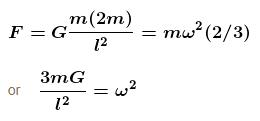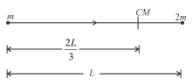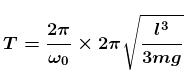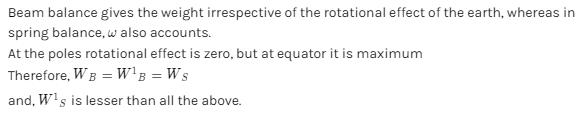Courses

# Central Forces MSQ

## 10 Questions MCQ Test Topic wise Tests for IIT JAM Physics | Central Forces MSQ

Description
This mock test of Central Forces MSQ for IIT JAM helps you for every IIT JAM entrance exam. This contains 10 Multiple Choice Questions for IIT JAM Central Forces MSQ (mcq) to study with solutions a complete question bank. The solved questions answers in this Central Forces MSQ quiz give you a good mix of easy questions and tough questions. IIT JAM students definitely take this Central Forces MSQ exercise for a better result in the exam. You can find other Central Forces MSQ extra questions, long questions & short questions for IIT JAM on EduRev as well by searching above.
*Multiple options can be correct
QUESTION: 1

### Three point masses, m each are at the corners of an equilateral triangle of side a. Their separations do not change when the system rotates about the centre of the triangle. For this, the time period of rotation must be proportional to :

Solution:

*Multiple options can be correct
QUESTION: 2

### A solid sphere of uniform density and radius 4units is located with its centre at the origin of coordinates, O. Two spheres of equal radii of 1unit, with their centres at A(-2,0,0) and B(2, 0, 0) respectively, are taken out of the solid sphere, leaving behind spherical cavities as shown in the figure.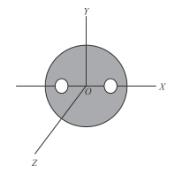Solution:

Use arguments of symmetry as the yz plane divides the objects symmetrically.

The correct answers are: The gravitational force due to this object at the origin is zero, The gravitational potential is the same at all points of the circle y2 + z2 = 36, The gravitational potential is the same at all points of the circle y2 + z2 = 4

*Multiple options can be correct
QUESTION: 3

### A satellite revolves around a planet in circular orbit of radius R (much larger than the radius of the planet) with a time period of revolution T. If the satellite is stopped and then released in its orbits (Assume that the satellite experiences gravitational force due to the planet only).

Solution:

It will fall because mg is acting on it towards the centre of planet and initial velocity is zero. It’ll move in straight line.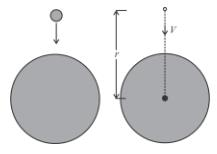By energy conservation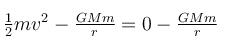using this we get  V = f(r)
Now use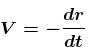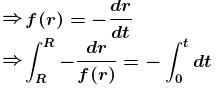R' = radius of the planet.
In the final expression (on in the beginning itself)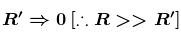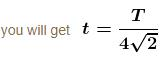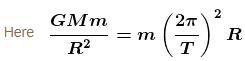The correct answers are: It will fall on the planet, The time of fall of the satellite on the planet is nearly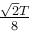*Multiple options can be correct
QUESTION: 4

Let V and E denotes the gravitational potential and gravitational field at the point. It is possible to have :

Solution:

A) At ∞ both V and E are zero.
B) Let, V​=GM/R​ for an unit mass.

So, VR​=0 i.e. at the radius R of solid sphere(mass M) and ER​=GM/R2
C) Inside a spherical shell V =GM/R​ and E =0
Thus, all the above are correct.

*Multiple options can be correct
QUESTION: 5

A small mass m is moved slowly from the surface of the earth to a height above the surface. The work done (by an external agent) in doing this is :

Solution:

For, h<<R force is constant and is equal to mg

Therefore, work done is mgh in moving by distance h

For, h=R

gravitational potential at x is − GM/x

Work done in moving from x=R to x=2R is − GMm/2R + GMm/R

​= GMm/2R

which is equal to  1/2 mgR

*Multiple options can be correct
QUESTION: 6

A satellite close to the earth is in orbit above the equator with the period of rotation of 1.5hours. If it is above a point P on the equator at some time, it will be above P again after time :

Solution:

Let ω0 = the angular velocity of the earth about its axis.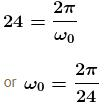Let ω = the angular velocity of the satellite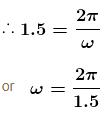For a satellite rotating from west to east (the same as the earth), the relative angular velocity, ω1 = ω - ω0
The period of rotation relative to the earth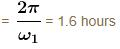For a satellite rotating from east to west (opposite to the earth), the relative angular velocity,  ω2 = ω + ω0.
The correct answers are: 1.6hours if it is rotating from west to east, 24/17hours if it is rotating from east to west

*Multiple options can be correct
QUESTION: 7

The magnitudes of the gravitational field at distance r1 and  r2 from the centre of a uniform sphere of radius R and mass M are  F1 and  F2 respectively. Then :

Solution:

F ∝ T,  if r < R, and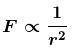if r > R.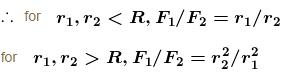The correct answers are: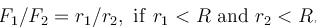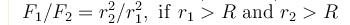*Multiple options can be correct
QUESTION: 8

A double star is a system of two stars of masses m and 2m, rotating about their centre of mass only under their mutual gravitational attraction. If r is the separation between these two stars then their time period of rotation about their centre of mass will be proportional to :

Solution: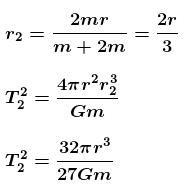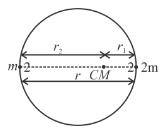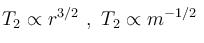*Multiple options can be correct
QUESTION: 9

A binary star is a system of two stars rotating about their centre of mass only under their mutual gravitational attraction. Let the stars have masses m and  2and let their separation be l. Their time period of rotation about their centre of mass will be proportional to :

Solution: